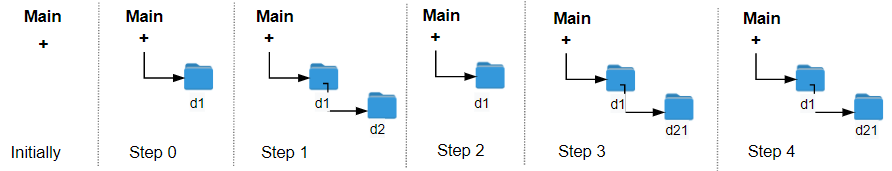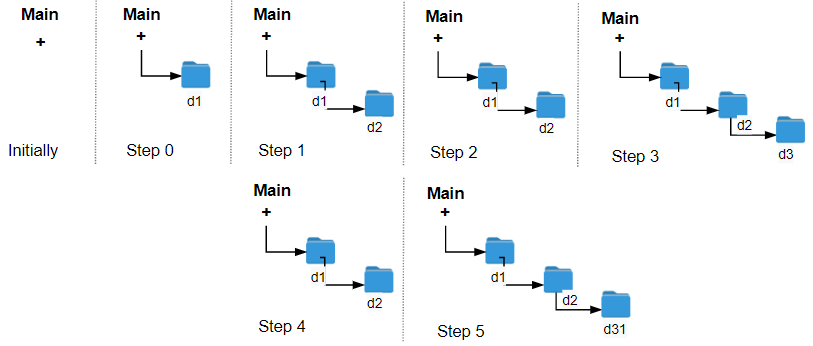# GeetCode Hub

The Leetcode file system keeps a log each time some user performs a change folder operation.

The operations are described below:

• `"../"` : Move to the parent folder of the current folder. (If you are already in the main folder, remain in the same folder).
• `"./"` : Remain in the same folder.
• `"x/"` : Move to the child folder named `x` (This folder is guaranteed to always exist).

You are given a list of strings `logs` where `logs[i]` is the operation performed by the user at the `ith` step.

The file system starts in the main folder, then the operations in `logs` are performed.

Return the minimum number of operations needed to go back to the main folder after the change folder operations.

Example 1:```Input: logs = ["d1/","d2/","../","d21/","./"]
Output: 2
Explanation: Use this change folder operation "../" 2 times and go back to the main folder.
```

Example 2:```Input: logs = ["d1/","d2/","./","d3/","../","d31/"]
Output: 3
```

Example 3:

```Input: logs = ["d1/","../","../","../"]
Output: 0
```

Constraints:

• `1 <= logs.length <= 103`
• `2 <= logs[i].length <= 10`
• `logs[i]` contains lowercase English letters, digits, `'.'`, and `'/'`.
• `logs[i]` follows the format described in the statement.
• Folder names consist of lowercase English letters and digits.

public class Solution { public int MinOperations(string[] logs) { } }